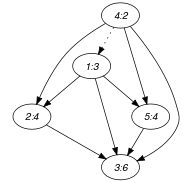## 5.130. distance_between

Origin

N. Beldiceanu

Constraint

$\mathrm{𝚍𝚒𝚜𝚝𝚊𝚗𝚌𝚎}_\mathrm{𝚋𝚎𝚝𝚠𝚎𝚎𝚗}\left(\mathrm{𝙳𝙸𝚂𝚃},\mathrm{𝚅𝙰𝚁𝙸𝙰𝙱𝙻𝙴𝚂}\mathtt{1},\mathrm{𝚅𝙰𝚁𝙸𝙰𝙱𝙻𝙴𝚂}\mathtt{2},\mathrm{𝙲𝚃𝚁}\right)$

Synonym

$\mathrm{𝚍𝚒𝚜𝚝𝚊𝚗𝚌𝚎}$.

Arguments
 $\mathrm{𝙳𝙸𝚂𝚃}$ $\mathrm{𝚍𝚟𝚊𝚛}$ $\mathrm{𝚅𝙰𝚁𝙸𝙰𝙱𝙻𝙴𝚂}\mathtt{1}$ $\mathrm{𝚌𝚘𝚕𝚕𝚎𝚌𝚝𝚒𝚘𝚗}\left(\mathrm{𝚟𝚊𝚛}-\mathrm{𝚍𝚟𝚊𝚛}\right)$ $\mathrm{𝚅𝙰𝚁𝙸𝙰𝙱𝙻𝙴𝚂}\mathtt{2}$ $\mathrm{𝚌𝚘𝚕𝚕𝚎𝚌𝚝𝚒𝚘𝚗}\left(\mathrm{𝚟𝚊𝚛}-\mathrm{𝚍𝚟𝚊𝚛}\right)$ $\mathrm{𝙲𝚃𝚁}$ $\mathrm{𝚊𝚝𝚘𝚖}$
Restrictions
 $\mathrm{𝙳𝙸𝚂𝚃}\ge 0$ $\mathrm{𝙳𝙸𝚂𝚃}\le |\mathrm{𝚅𝙰𝚁𝙸𝙰𝙱𝙻𝙴𝚂}\mathtt{1}|*|\mathrm{𝚅𝙰𝚁𝙸𝙰𝙱𝙻𝙴𝚂}\mathtt{2}|-|\mathrm{𝚅𝙰𝚁𝙸𝙰𝙱𝙻𝙴𝚂}\mathtt{1}|$ $\mathrm{𝚛𝚎𝚚𝚞𝚒𝚛𝚎𝚍}$$\left(\mathrm{𝚅𝙰𝚁𝙸𝙰𝙱𝙻𝙴𝚂}\mathtt{1},\mathrm{𝚟𝚊𝚛}\right)$ $\mathrm{𝚛𝚎𝚚𝚞𝚒𝚛𝚎𝚍}$$\left(\mathrm{𝚅𝙰𝚁𝙸𝙰𝙱𝙻𝙴𝚂}\mathtt{2},\mathrm{𝚟𝚊𝚛}\right)$ $|\mathrm{𝚅𝙰𝚁𝙸𝙰𝙱𝙻𝙴𝚂}\mathtt{1}|=|\mathrm{𝚅𝙰𝚁𝙸𝙰𝙱𝙻𝙴𝚂}\mathtt{2}|$ $\mathrm{𝙲𝚃𝚁}\in \left[=,\ne ,<,\ge ,>,\le \right]$
Purpose

Let ${U}_{i}$ and ${V}_{i}$ be respectively the ${i}^{th}$ and ${j}^{th}$ variables $\left(i\ne j\right)$ of the collection $\mathrm{𝚅𝙰𝚁𝙸𝙰𝙱𝙻𝙴𝚂}\mathtt{1}$. In a similar way, let ${X}_{i}$ and ${Y}_{i}$ be respectively the ${i}^{th}$ and ${j}^{th}$ variables $\left(i\ne j\right)$ of the collection $\mathrm{𝚅𝙰𝚁𝙸𝙰𝙱𝙻𝙴𝚂}\mathtt{2}$. $\mathrm{𝙳𝙸𝚂𝚃}$ is equal to the number of times one of the following mutually incompatible conditions are true:

• ${U}_{i}\mathrm{𝙲𝚃𝚁}{V}_{i}$ holds and ${X}_{i}\mathrm{𝙲𝚃𝚁}{Y}_{i}$ does not hold,

• ${X}_{i}\mathrm{𝙲𝚃𝚁}{Y}_{i}$ holds and ${U}_{i}\mathrm{𝙲𝚃𝚁}{V}_{i}$ does not hold.

Example
$\left(2,〈3,4,6,2,4〉,〈2,6,9,3,6〉,<\right)$

The $\mathrm{𝚍𝚒𝚜𝚝𝚊𝚗𝚌𝚎}_\mathrm{𝚋𝚎𝚝𝚠𝚎𝚎𝚗}$ constraint holds since the following $\mathrm{𝙳𝙸𝚂𝚃}=2$ conditions are verified:

• $\begin{array}{c}\mathrm{𝚅𝙰𝚁𝙸𝙰𝙱𝙻𝙴𝚂}\mathtt{1}\left[4\right].\mathrm{𝚟𝚊𝚛}=2<\mathrm{𝚅𝙰𝚁𝙸𝙰𝙱𝙻𝙴𝚂}\mathtt{1}\left[1\right].\mathrm{𝚟𝚊𝚛}=3\wedge \hfill \\ \mathrm{𝚅𝙰𝚁𝙸𝙰𝙱𝙻𝙴𝚂}\mathtt{2}\left[4\right].\mathrm{𝚟𝚊𝚛}=3\ge \mathrm{𝚅𝙰𝚁𝙸𝙰𝙱𝙻𝙴𝚂}\mathtt{2}\left[1\right].\mathrm{𝚟𝚊𝚛}=2\hfill \end{array}$

• $\begin{array}{c}\mathrm{𝚅𝙰𝚁𝙸𝙰𝙱𝙻𝙴𝚂}\mathtt{2}\left[1\right].\mathrm{𝚟𝚊𝚛}=2<\mathrm{𝚅𝙰𝚁𝙸𝙰𝙱𝙻𝙴𝚂}\mathtt{2}\left[4\right].\mathrm{𝚟𝚊𝚛}=3\wedge \hfill \\ \mathrm{𝚅𝙰𝚁𝙸𝙰𝙱𝙻𝙴𝚂}\mathtt{1}\left[1\right].\mathrm{𝚟𝚊𝚛}=3\ge \mathrm{𝚅𝙰𝚁𝙸𝙰𝙱𝙻𝙴𝚂}\mathtt{1}\left[4\right].\mathrm{𝚟𝚊𝚛}=2\hfill \end{array}$

Typical
 $\mathrm{𝙳𝙸𝚂𝚃}>0$ $\mathrm{𝙳𝙸𝚂𝚃}<|\mathrm{𝚅𝙰𝚁𝙸𝙰𝙱𝙻𝙴𝚂}\mathtt{1}|*|\mathrm{𝚅𝙰𝚁𝙸𝙰𝙱𝙻𝙴𝚂}\mathtt{2}|-|\mathrm{𝚅𝙰𝚁𝙸𝙰𝙱𝙻𝙴𝚂}\mathtt{1}|$ $|\mathrm{𝚅𝙰𝚁𝙸𝙰𝙱𝙻𝙴𝚂}\mathtt{1}|>1$ $\mathrm{𝙲𝚃𝚁}\in \left[=,\ne \right]$
Symmetries
• Arguments are permutable w.r.t. permutation $\left(\mathrm{𝙳𝙸𝚂𝚃}\right)$ $\left(\mathrm{𝚅𝙰𝚁𝙸𝙰𝙱𝙻𝙴𝚂}\mathtt{1},\mathrm{𝚅𝙰𝚁𝙸𝙰𝙱𝙻𝙴𝚂}\mathtt{2}\right)$ $\left(\mathrm{𝙲𝚃𝚁}\right)$.

• Items of $\mathrm{𝚅𝙰𝚁𝙸𝙰𝙱𝙻𝙴𝚂}\mathtt{1}$ and $\mathrm{𝚅𝙰𝚁𝙸𝙰𝙱𝙻𝙴𝚂}\mathtt{2}$ are permutable (same permutation used).

• One and the same constant can be added to the $\mathrm{𝚟𝚊𝚛}$ attribute of all items of $\mathrm{𝚅𝙰𝚁𝙸𝙰𝙱𝙻𝙴𝚂}\mathtt{1}$.

• One and the same constant can be added to the $\mathrm{𝚟𝚊𝚛}$ attribute of all items of $\mathrm{𝚅𝙰𝚁𝙸𝙰𝙱𝙻𝙴𝚂}\mathtt{2}$.

Arg. properties

Functional dependency: $\mathrm{𝙳𝙸𝚂𝚃}$ determined by $\mathrm{𝚅𝙰𝚁𝙸𝙰𝙱𝙻𝙴𝚂}\mathtt{1}$, $\mathrm{𝚅𝙰𝚁𝙸𝙰𝙱𝙻𝙴𝚂}\mathtt{2}$ and $\mathrm{𝙲𝚃𝚁}$.

Usage

Measure the distance between two sequences in term of the number of constraint changes. This should be put in contrast to the number of value changes that is sometimes superficial.

Keywords
Arc input(s)

$\mathrm{𝚅𝙰𝚁𝙸𝙰𝙱𝙻𝙴𝚂}\mathtt{1}$/ $\mathrm{𝚅𝙰𝚁𝙸𝙰𝙱𝙻𝙴𝚂}\mathtt{2}$

Arc generator
$\mathrm{𝐶𝐿𝐼𝑄𝑈𝐸}$$\left(\ne \right)↦\mathrm{𝚌𝚘𝚕𝚕𝚎𝚌𝚝𝚒𝚘𝚗}\left(\mathrm{𝚟𝚊𝚛𝚒𝚊𝚋𝚕𝚎𝚜}\mathtt{1},\mathrm{𝚟𝚊𝚛𝚒𝚊𝚋𝚕𝚎𝚜}\mathtt{2}\right)$

Arc arity
Arc constraint(s)
$\mathrm{𝚟𝚊𝚛𝚒𝚊𝚋𝚕𝚎𝚜}\mathtt{1}.\mathrm{𝚟𝚊𝚛}\mathrm{𝙲𝚃𝚁}\mathrm{𝚟𝚊𝚛𝚒𝚊𝚋𝚕𝚎𝚜}\mathtt{2}.\mathrm{𝚟𝚊𝚛}$
Graph property(ies)
$\mathrm{𝐃𝐈𝐒𝐓𝐀𝐍𝐂𝐄}$$=\mathrm{𝙳𝙸𝚂𝚃}$

Graph model

Within the Arc input(s) slot, the character / indicates that we generate two distinct graphs. The graph property $\mathrm{𝐃𝐈𝐒𝐓𝐀𝐍𝐂𝐄}$ measures the distance between two digraphs ${G}_{1}$ and ${G}_{2}$. This distance is defined as the sum of the following quantities:

• The number of arcs of ${G}_{1}$ that do not belong to ${G}_{2}$,

• The number of arcs of ${G}_{2}$ that do not belong to ${G}_{1}$.

Part (A) of Figure 5.130.1 gives the final graph associated with the sequence $\mathrm{𝚟𝚊𝚛}$-3,$\mathrm{𝚟𝚊𝚛}$-4,$\mathrm{𝚟𝚊𝚛}$-6,$\mathrm{𝚟𝚊𝚛}$-2,$\mathrm{𝚟𝚊𝚛}$-4 (i.e., the second argument of the constraint of the Example slot), while part (B) shows the final graph corresponding to $\mathrm{𝚟𝚊𝚛}$-2,$\mathrm{𝚟𝚊𝚛}$-6,$\mathrm{𝚟𝚊𝚛}$-9,$\mathrm{𝚟𝚊𝚛}$-3,$\mathrm{𝚟𝚊𝚛}$-6 (i.e., the third argument of the constraint of the Example slot). The two arc constraints that differ from one graph to the other are marked by a dotted line. The $\mathrm{𝚍𝚒𝚜𝚝𝚊𝚗𝚌𝚎}_\mathrm{𝚋𝚎𝚝𝚠𝚎𝚎𝚗}$ constraint holds since between sequence $\mathrm{𝚟𝚊𝚛}$-3,$\mathrm{𝚟𝚊𝚛}$-4,$\mathrm{𝚟𝚊𝚛}$-6,$\mathrm{𝚟𝚊𝚛}$-2,$\mathrm{𝚟𝚊𝚛}$-4 and sequence $\mathrm{𝚟𝚊𝚛}$-2,$\mathrm{𝚟𝚊𝚛}$-6,$\mathrm{𝚟𝚊𝚛}$-9,$\mathrm{𝚟𝚊𝚛}$-3,$\mathrm{𝚟𝚊𝚛}$-6 there are $\mathrm{𝙳𝙸𝚂𝚃}=2$ changes that respectively correspond to:

• Within the final graph associated with sequence $\mathrm{𝚟𝚊𝚛}$-3,$\mathrm{𝚟𝚊𝚛}$-4,$\mathrm{𝚟𝚊𝚛}$-6,$\mathrm{𝚟𝚊𝚛}$-2,$\mathrm{𝚟𝚊𝚛}$-4 the arc $4\to 1$ (i.e., values $2\to 3$) does not occur in the final graph associated with $\mathrm{𝚟𝚊𝚛}$-2,$\mathrm{𝚟𝚊𝚛}$-6,$\mathrm{𝚟𝚊𝚛}$-9,$\mathrm{𝚟𝚊𝚛}$-3,$\mathrm{𝚟𝚊𝚛}$-6,

• Within the final graph associated with sequence $\mathrm{𝚟𝚊𝚛}$-2,$\mathrm{𝚟𝚊𝚛}$-6,$\mathrm{𝚟𝚊𝚛}$-9,$\mathrm{𝚟𝚊𝚛}$-3,$\mathrm{𝚟𝚊𝚛}$-6 the arc $1\to 4$ (i.e., values $2\to 3$) does not occur in the final graph associated with $\mathrm{𝚟𝚊𝚛}$-3,$\mathrm{𝚟𝚊𝚛}$-4,$\mathrm{𝚟𝚊𝚛}$-6,$\mathrm{𝚟𝚊𝚛}$-2,$\mathrm{𝚟𝚊𝚛}$-4.

##### Figure 5.130.1. Final graphs of the $\mathrm{𝚍𝚒𝚜𝚝𝚊𝚗𝚌𝚎}_\mathrm{𝚋𝚎𝚝𝚠𝚎𝚎𝚗}$ constraint(a) (b)